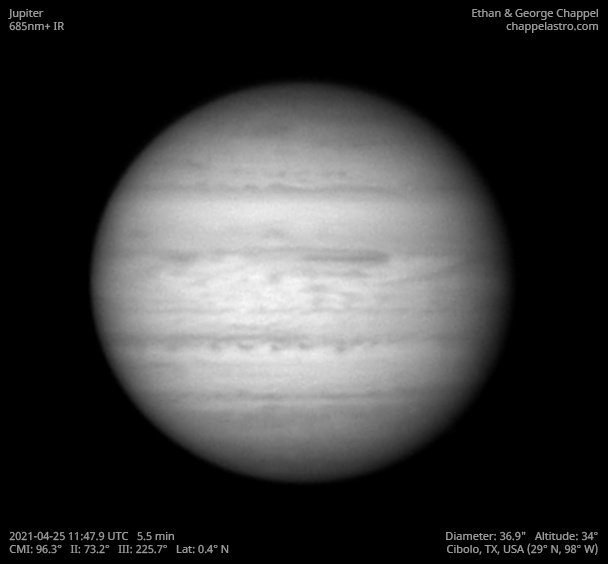# Jupiter 2021-04-25 11:47 UTC

CM1: 96.30°

CM2: 73.20°

CM3: 225.70°

CLat: +0.40°

Description

Sky conditions were significantly better than two weeks ago. Jupiter is now reaching 30 degrees high while it is dark enough to image. It will transit 47 degrees from my location this apparition, about 9 degrees higher than last year.

The North and South Equatorial Belt both appear faded, except for the southern edge of the NEB. The Equatorial Zone is intensely orange. The Great Red Spot is setting on the right.

Equipment

ZWO ASI290MM

Celestron EdgeHD 14

Logs
```FireCapture v2.7 04 BETA Settings
------------------------------------
Observer=Ethan Chappel
Location=Cibolo
Scope=Celestron C8 EdgeHD
Camera=ZWO ASI290MM
Filter=IR685
Profile=Jupiter
Diameter=36.80"
Magnitude=-2.19
CMI=94.8° CMII=71.8° CMIII=224.2°  (during mid of capture)
FocalLength=6550mm (F/32)
Resolution=0.09"
Filename=j2021-04-25_11-46-25_ir685_ec.ser
Date=2021_04_25
Start=11_45_10.540
Mid=11_46_25.543
End=11_47_40.547
Start(UT)=11_45_10.540
Mid(UT)=11_46_25.543
End(UT)=11_47_40.547
Duration=150.007s
Date_format=yyyy_MM_dd
Time_format=HH_mm_ss
LT=UT
Frames captured=7160
File type=SER
Binning=1x1
ROI=556x546
ROI(Offset)=920x24
FPS (avg.)=47
Shutter=12.00ms
Gain=320 (53%)
AutoExposure=off
AutoGain=off
Brightness=1
HighSpeed=off
USBTraffic=50
Histogramm(min)=0
Histogramm(max)=160
Histogramm=62%
Noise(avg.deviation)=4.20
Limit=150 Seconds
Sensor temperature=76.5°F
Focuser position=1350
FireCapture v2.7 04 BETA Settings
------------------------------------
Observer=Ethan Chappel
Location=Cibolo
Scope=Celestron C8 EdgeHD
Camera=ZWO ASI290MM
Filter=IR685
Profile=Jupiter
Diameter=36.81"
Magnitude=-2.19
CMI=96.6° CMII=73.5° CMIII=226.0°  (during mid of capture)
FocalLength=6350mm (F/31)
Resolution=0.09"
Filename=j2021-04-25_11-49-22_ir685_ec.ser
Date=2021_04_25
Start=11_48_07.355
Mid=11_49_22.363
End=11_50_37.372
Start(UT)=11_48_07.355
Mid(UT)=11_49_22.363
End(UT)=11_50_37.372
Duration=150.017s
Date_format=yyyy_MM_dd
Time_format=HH_mm_ss
LT=UT
Frames captured=7160
File type=SER
Binning=1x1
ROI=556x546
ROI(Offset)=920x24
FPS (avg.)=47
Shutter=12.00ms
Gain=320 (53%)
AutoExposure=off
AutoGain=off
Brightness=1
HighSpeed=off
USBTraffic=50
Histogramm(min)=2
Histogramm(max)=129
Histogramm=50%
Noise(avg.deviation)=4.76
Limit=150 Seconds
Sensor temperature=76.6°F
Focuser position=1370
```